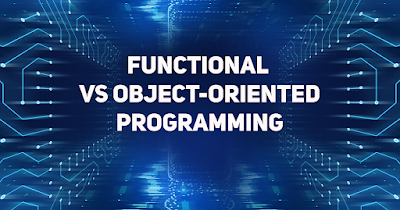# Imperative vs Functional vs Object-oriented Programming

0

Imperative vs Functional vs Object-oriented ProgrammingImperative vs Functional vs Object-oriented Programming

## 1. Imperative Programming:

Definition:

• Imperative programming is a paradigm where the program consists of a sequence of statements that change the program's state.

Key Characteristics:

• Uses statements that change a program's state.
• Emphasizes how to perform a task.
• Common in languages like C, Pascal, and Fortran.

Example:

```# Imperative Python code
total = 0
for num in range(1, 6):
total += num
print(total)
```

### 2. Functional Programming:

Definition:

• Functional programming is a paradigm that treats computation as the evaluation of mathematical functions and avoids changing state and mutable data.

Key Characteristics:

• Avoids changing state and mutable data.
• Emphasizes expressions and declarations.
• Common in languages like Haskell, Lisp, and Scala.

Example:

```# Functional Python code
total = sum(range(1, 6))
print(total)
```

## 3. Object-oriented Programming (OOP):

Definition:

• Object-oriented programming is a paradigm where the program is organized around objects that represent real-world entities, and data and functions are encapsulated within these objects.

Key Characteristics:

• Organizes code into classes and objects.
• Emphasizes encapsulation, inheritance, and polymorphism.
• Common in languages like Java, Python, and C++.

Example:

```# Object-oriented Python code
class Summation:
def __init__(self):
self.total = 0

self.total = sum(nums)

# Creating an object
summation_obj = Summation()
print(summation_obj.total)

```

## 4. Comparison:

1. State Management:

• Imperative: Focuses on changing state.
• Functional: Avoids changing state, emphasizes immutability.
• Object-oriented: Organizes state within objects.

• Imperative: How to perform a task.
• Functional: What to perform; emphasizes expressions.
• Object-oriented: Organizes code around objects and their interactions.

3. Code Structure:

• Imperative: Uses statements and procedures.
• Functional: Uses functions and expressions.
• Object-oriented: Uses classes and objects.

4. Common Languages:

• Imperative: C, Fortran, Pascal.
• Object-oriented: Java, Python, C++.

5. Pros and Cons:

• Imperative: Explicit, but can lead to complex code.
• Functional: Promotes clear and concise code but has a learning curve.
• Object-oriented: Encourages modularity and reuse but can be more verbose.

Choosing a programming paradigm often depends on the problem domain, team expertise, and specific project requirements. Many modern languages support multiple paradigms, allowing developers to choose the most suitable approach for a given task.